Question

# Exercise 1.16. We flip a fair coin five times

Exercise 1.16. We flip a fair coin five times. For every heads you pay me \$1 and for every tails I pay you \$1. Let X denote my net winnings at the end of five flips. Find the possible values and the probability mass function of X.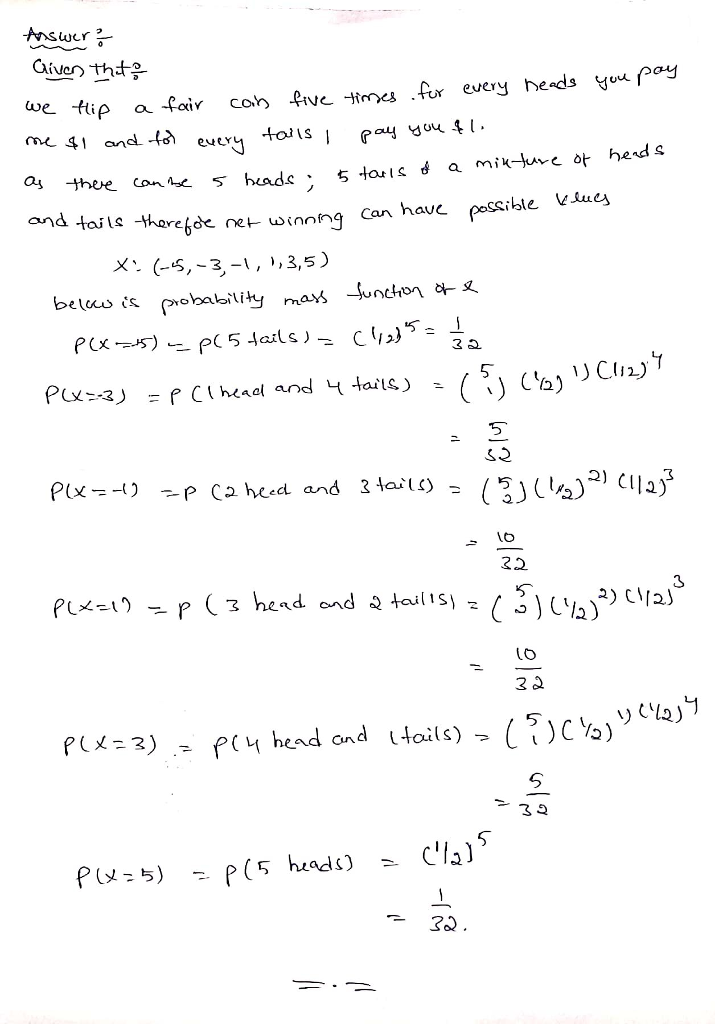#### Earn Coins

Coins can be redeemed for fabulous gifts.

Similar Homework Help Questions
• ### Exercise 10.17. We flip a fair coin. If it is heads we roll 3 dice. If...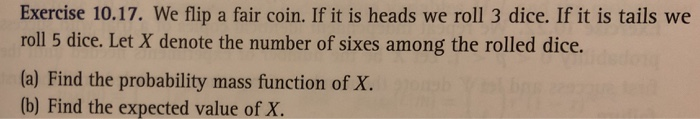Exercise 10.17. We flip a fair coin. If it is heads we roll 3 dice. If it is tails we roll 5 dice. Let X denote the number of sixes among the rolled dice. (a) Find the probability mass function of X. (b) Find the expected value of X.

• ### We flip a fair coin 10 times. What is the probability that there are at least...

We flip a fair coin 10 times. What is the probability that there are at least 4 heads out of the 10 flips?

• ### Suppose we flip a fair coin n times. We say that the sequence is balanced when there are equal number of heads and tails...

Suppose we flip a fair coin n times. We say that the sequence is balanced when there are equal number of heads and tails. For example, if we flip the coin 10 times and the results are HT HHT HT T HH, then this sequence balanced 2 times, i.e. at position 2 and position 8 (after the second and eighth flips). In terms of n, what is the expected number of times the sequence is balanced within n flips?

• ### Exercise 8.52. A fair coin is flipped 30 times. LetX denote the number of heads among...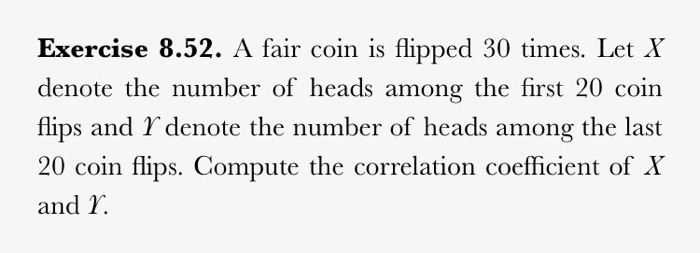Exercise 8.52. A fair coin is flipped 30 times. LetX denote the number of heads among the first 20 coin flips and Y denote the number of heads among the last 20 coin flips. Compute the correlation coefficient of X and I.

• ### Would you be more likely to get at least 70% tails if you flip a fair coin 10 times or if you flip a fair coin 1000 times

Would you be more likely to get at least 70% tails if you flip a fair coin 10 times or if you flip a faircoin 1000 times?A) You would be more likely to get at least 70% tails if you flip a fair coin 10 times than if youflip a fair coin 1000 times.B) The probability of getting at least 70% tails would be the same regardless of whether the coinis flipped 10 or 1000 times.C) You would be more...

• ### Question 2 Suppose you have a fair coin (a coin is considered fair if there is...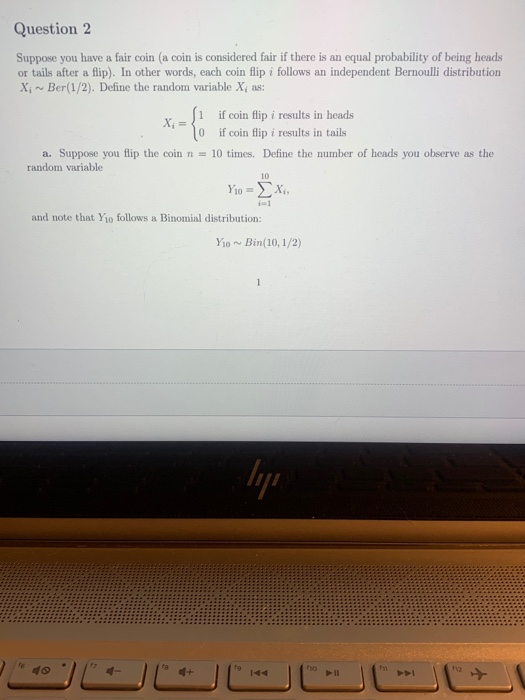Question 2 Suppose you have a fair coin (a coin is considered fair if there is an equal probability of being heads or tails after a flip). In other words, each coin flip i follows an independent Bernoulli distribution X Ber(1/2). Define the random variable X, as: i if coin flip i results in heads 10 if coin flip i results in tails a. Suppose you flip the coin n = 10 times. Define the number of heads you observe...

• ### 1. Multiple choice. Circle all the correct answers a) You flip a coin 100,000 times and...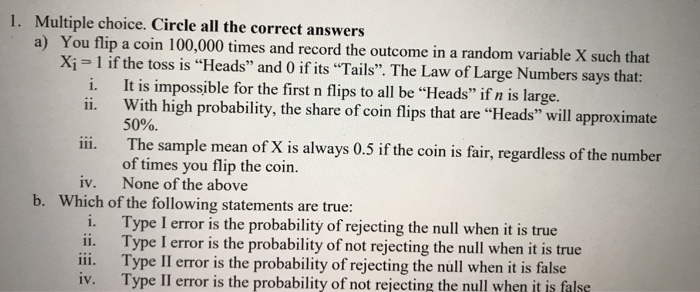1. Multiple choice. Circle all the correct answers a) You flip a coin 100,000 times and record the outcome in a Xi 1 if the toss is "Heads" and 0 if its "Tails. The Law of Large Numbers says that: i. ii. It is impossible for the first n flips to all be "Heads" if n is large. With high probability, the share of coin flips that are "Heads" will approximate 50%. The sample mean of X is always 0.5...

• ### You flip the same coin 90 mores times (100 total flips). If half of the 90...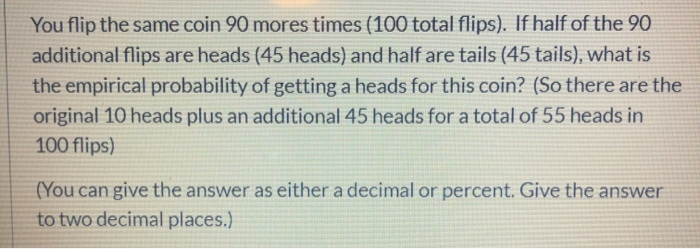You flip the same coin 90 mores times (100 total flips). If half of the 90 additional flips are heads (45 heads) and half are tails (45 tails), what is the empirical probability of getting a heads for this coin? (So there are the original 10 heads plus an additional 45 heads for a total of 55 heads in 100 flips) (You can give the answer as either a decimal or percent. Give the answer to two decimal places.)

• ### Flip a coin 10 times and record the observed number of heads and tails. For example,...

Flip a coin 10 times and record the observed number of heads and tails. For example, with 10 flips one might get 6 heads and 4 tails. Now, flip the coin another 20 times (so 30 times in total) and again, record the observed number of heads and tails. Finally, flip the coin another 70 times (so 100 times in total) and record your results again. We would expect that the distribution of heads and tails to be 50/50. How...

• ### Suppose you flip a fair coin repeatedly until you see a Heads followed by another Heads...

Suppose you flip a fair coin repeatedly until you see a Heads followed by another Heads or a Tails followed by another Tails (i.e. until you see the pattern HH or TT). (a)What is the expected number of flips you need to make? (b)Suppose you repeat the above with a weighted coin that has probability of landing Heads equal to p.Show that the expected number of flips you need is 2+p(1−p)/1−p(1−p)